# Day 7: gghalves

Welcome back for the 7th day of the #packagecalendar, today we will continue our look at the billboards data from yesterday. The package of the day is gghalves created by Frederik Tiedemann.

``install.packages("gghalves")``

we will be working with Santa’s `elf` dataset. The data includes 50 observations for 3 elves (150 observations total) taken monthly describing the elfly capacities. Units have been removed due to being classified.

``````library(skimr)
skim(elf)``````
 Name elf Number of rows 150 Number of columns 5 _______________________ Column type frequency: factor 1 numeric 4 ________________________ Group variables None

Variable type: factor

skim_variable n_missing complete_rate ordered n_unique top_counts
Elf 0 1 FALSE 3 Bud: 50, Twi: 50, Hol: 50

Variable type: numeric

skim_variable n_missing complete_rate mean sd p0 p25 p50 p75 p100 hist
Toy Making Intensity 0 1 5.84 0.83 4.3 5.1 5.80 6.4 7.9 ▆▇▇▅▂
Sugar Consumption 0 1 3.06 0.44 2.0 2.8 3.00 3.3 4.4 ▁▆▇▂▁
Jolliness 0 1 3.76 1.77 1.0 1.6 4.35 5.1 6.9 ▇▁▆▇▂
Reindeer Training 0 1 1.20 0.76 0.1 0.3 1.30 1.8 2.5 ▇▁▇▅▃

since we have a categorical variable (`elf`) and a couple of continuous variables we could use ggplot2 to visualize the distributions. Let us take a first look at the TMI (Toy Making Intensity) variable. We can create a boxplot for each elf by using `geom_boxplot()`

``````library(ggplot2)
ggplot(elf, aes(Elf, `Toy Making Intensity`)) +
geom_boxplot()``````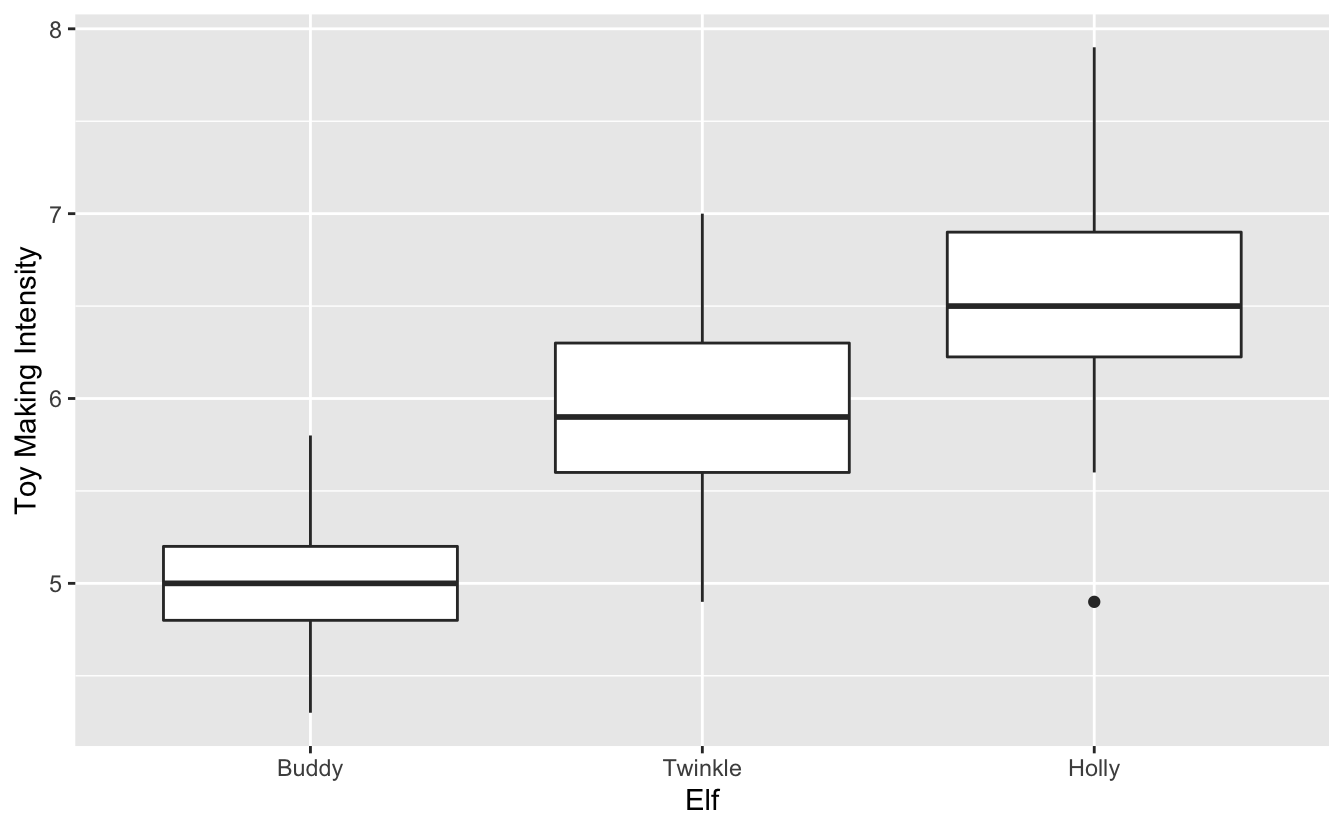But we can’t see the individual points. you could do a second plot with `geom_dotplot()`

``````ggplot(elf, aes(Elf, `Toy Making Intensity`)) +
geom_dotplot(binaxis = "y", stackdir = "center", binwidth = 0.05)``````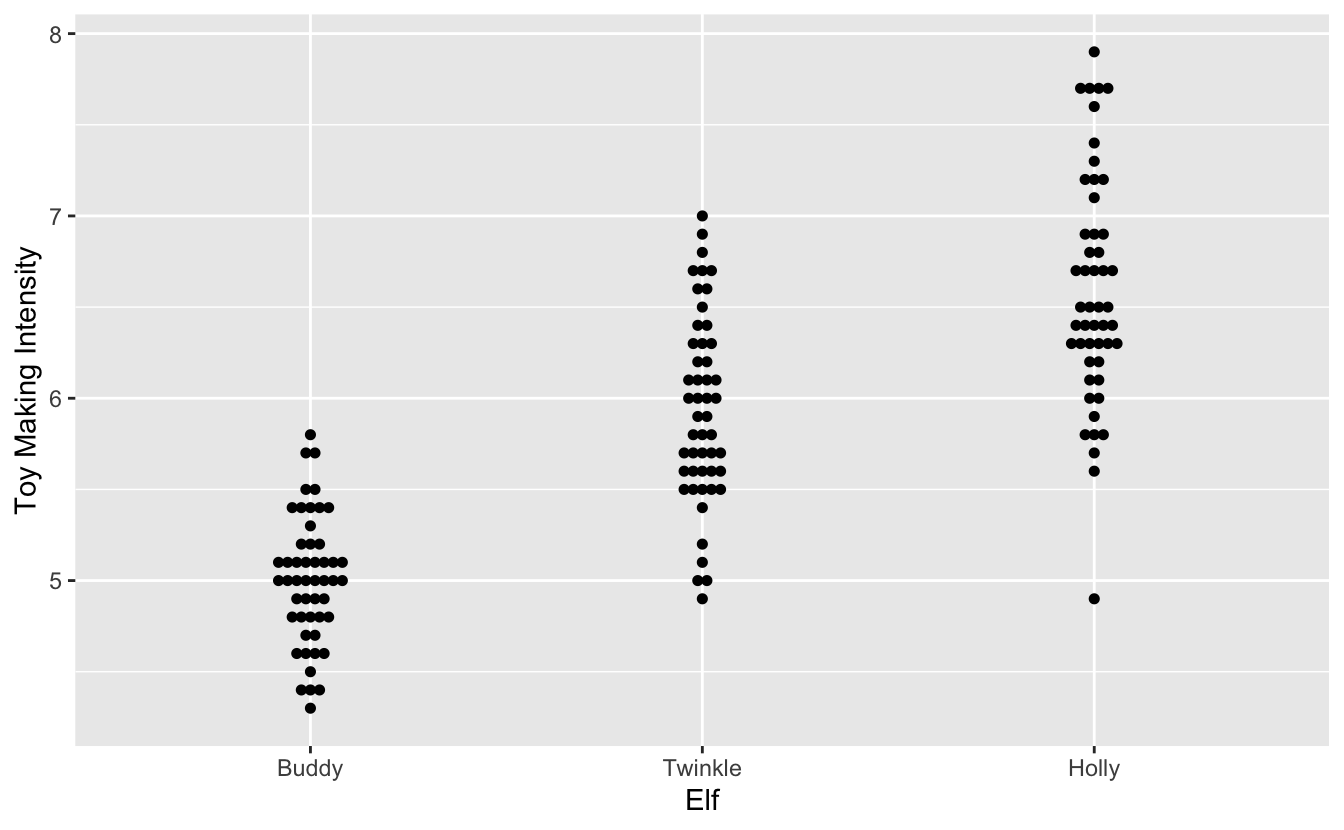But now we have two separate charts trying to show the same data. This is where gghalves comes in! gghalves allows you to split many of the aggregation geoms in half. It is easier to show with an example. We have the data from before and we want to showcase a boxplot and a dotplot at the same time.

``````library(gghalves)
ggplot(elf, aes(Elf, `Toy Making Intensity`)) +
geom_half_boxplot() +
geom_half_dotplot(binwidth = 0.05)``````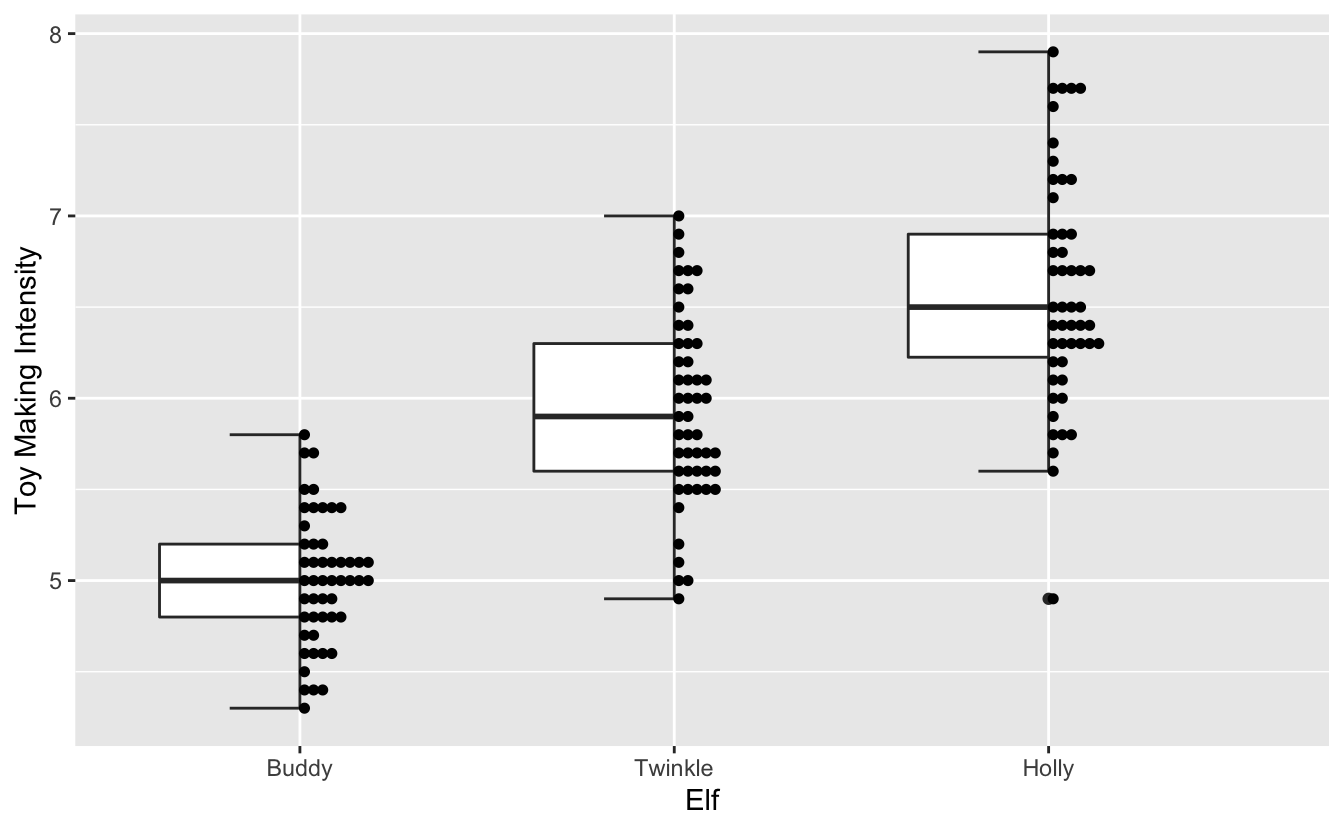by using `geom_half_boxplot()` and `geom_half_dotplot()` we we able to elegently combine 2 plot types.

The geoms respect general ggplot2 elements so things like color

``````ggplot(elf, aes(Elf, `Reindeer Training`, color = Elf)) +
geom_half_violin() +
geom_half_point(alpha = 0.6)``````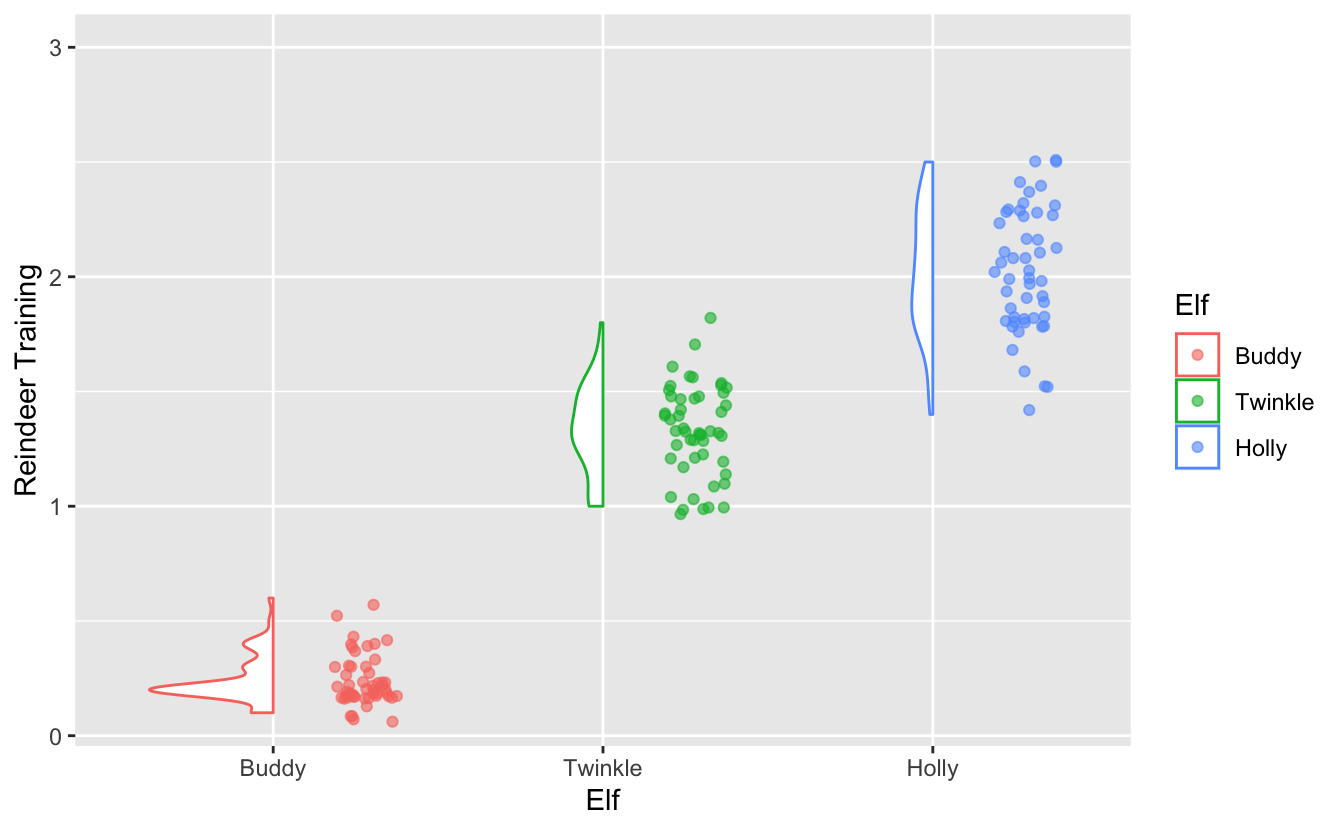and factors

``````ggplot(elf, aes(Elf, Jolliness, color = Elf)) +
geom_half_violin() +
geom_half_boxplot(side = "r") +
facet_wrap(~ factor(`Sugar Consumption` > mean(`Sugar Consumption`),
c(TRUE, FALSE),
c("High Sugar Comsumption", "Low Sugar Comsumption")))``````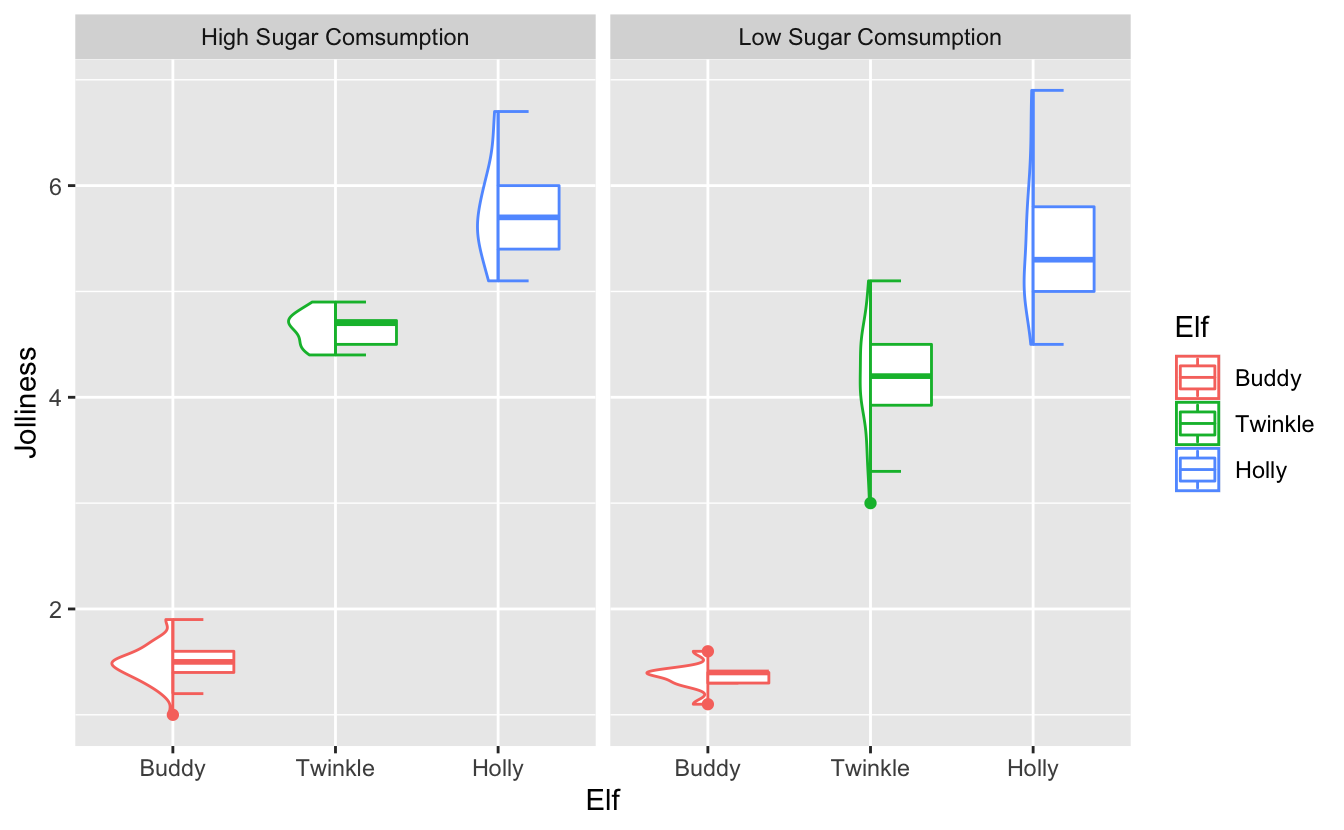Creating `elf` dataset

``````library(tidyverse)
set.seed(1234)

elf <- iris %>%
rename(Elf = Species,
`Sugar Consumption` = Sepal.Width,
`Toy Making Intensity` = Sepal.Length,
`Jolliness` = Petal.Length,
`Reindeer Training` = Petal.Width) %>%
mutate(Elf = factor(as.numeric(Elf), labels = c("Buddy", "Twinkle", "Holly"))) %>%
mutate_if(is.numeric, jitter)``````